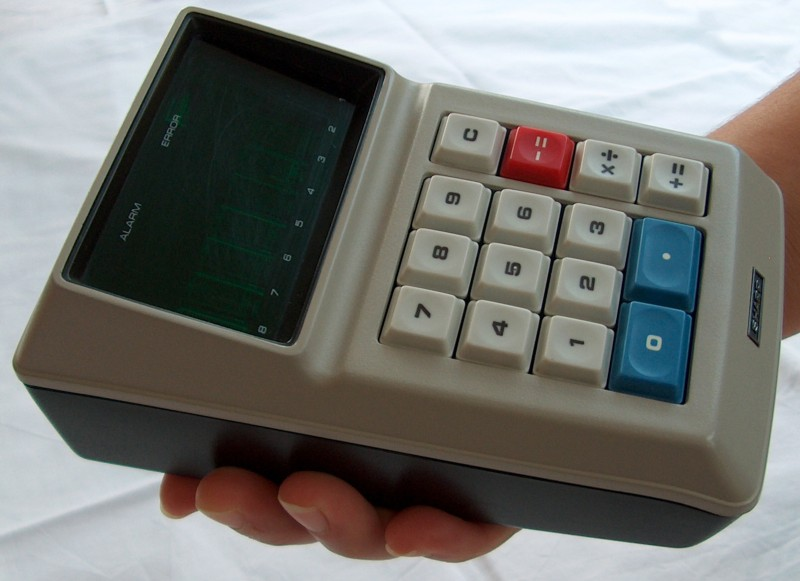Reverse engineering of the 1st Sharp calculator - Printable Version +- HP Forums (https://www.hpmuseum.org/forum) +-- Forum: Not HP Calculators (/forum-7.html) +--- Forum: Not remotely HP Calculators (/forum-9.html) +--- Thread: Reverse engineering of the 1st Sharp calculator (/thread-16077.html) Reverse engineering of the 1st Sharp calculator - celltx - 12-26-2020 08:03 PM An interesting piece of history: https://www.righto.com/2020/12/reverse-engineering-early-calculator.htmlRE: Reverse engineering of the 1st Sharp calculator - celltx - 12-27-2020 07:46 PM I wonder how did they use a combined multiply-divide button? Any ideas? RE: Reverse engineering of the 1st Sharp calculator - BruceH - 12-27-2020 08:53 PM (12-27-2020 07:46 PM)celltx Wrote:  I wonder how did they use a combined multiply-divide button? Any ideas? I think... enter first value, press [×÷], then second value then [+=] for multiply or [-=] for divide. RE: Reverse engineering of the 1st Sharp calculator - Steve Simpkin - 12-27-2020 09:02 PM (12-27-2020 08:53 PM)BruceH Wrote:   (12-27-2020 07:46 PM)celltx Wrote:  I wonder how did they use a combined multiply-divide button? Any ideas? I think... enter first value, press [×÷], then second value then [+=] for multiply or [-=] for divide. That is correct. "The QT-8D uses an unusual keyboard layout. Note the combined [X÷] key. Sharp used a novel means to save a key on the keyboard (thus saving the cost, as well as decreasing the real-estate needed for the keyboard) by making the [X÷] key serve both functions. The determination as to which function the user wishes is made by the user selecting either the [+=] key to generate the result for multiplication, and the [-=] key to cause division to occur. For example, to multiply, the user would enter the first number, press the [X÷] key, then enter the multiplier, then press the [+=] key for the answer. To divide, the user would enter the dividend, press the [X÷] key, enter the divisor, and press the [-=] key to calculate the answer." http://bensene.com/sharpqt-8d.html RE: Reverse engineering of the 1st Sharp calculator - Paul Dale - 12-28-2020 12:31 AM It could count as a very limited shift/prefix key. Like arc on the HP 35. Pauli